# Intermediate maths solutions for System of Circles

Intermediate mathematics IIA solutions for System of Circles exercises 2(a) and 2(b) are given.

Thses are very easy to understand. First you study the textbook lessons very well. Then observe the example problems and solutions. Try them.

Observe the given solutions and try them in your own method.

You can see solutions for Inter Maths IIB

1. Circle

3. Parabola

4. Ellipse

You can also see solutions for Inter Maths IIA

# Inter maths solutions for System of Circles

Exercise 2(a)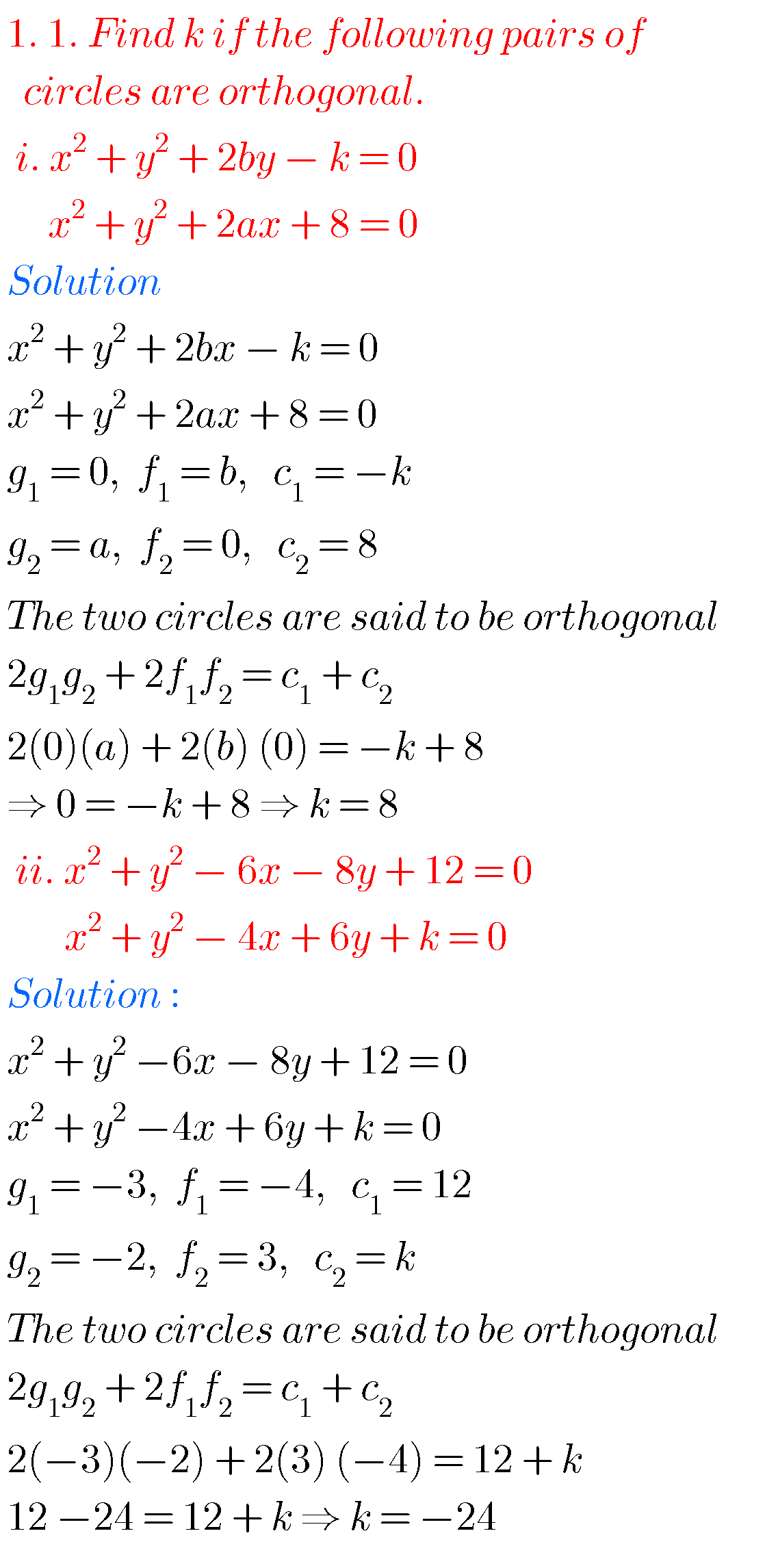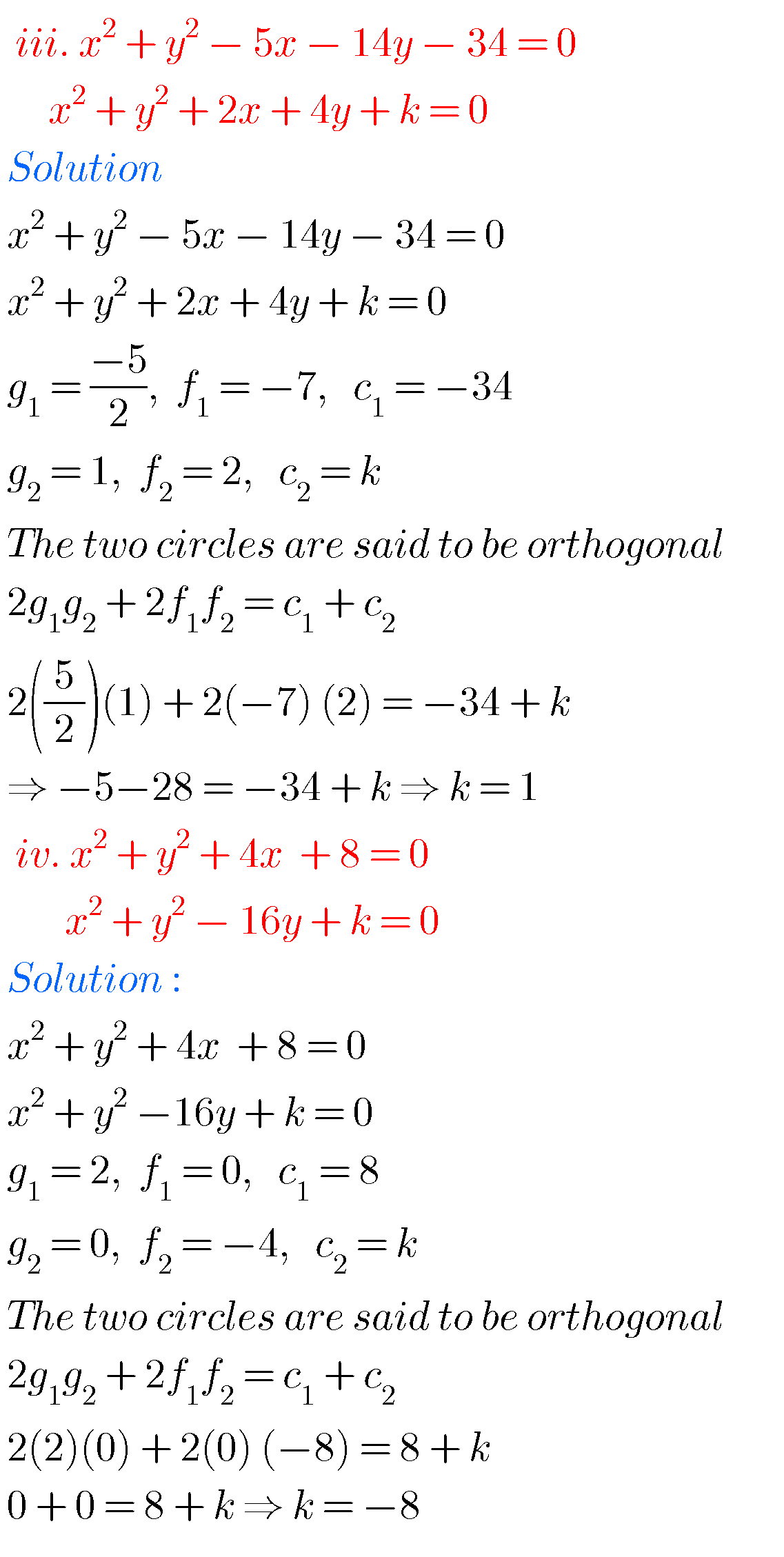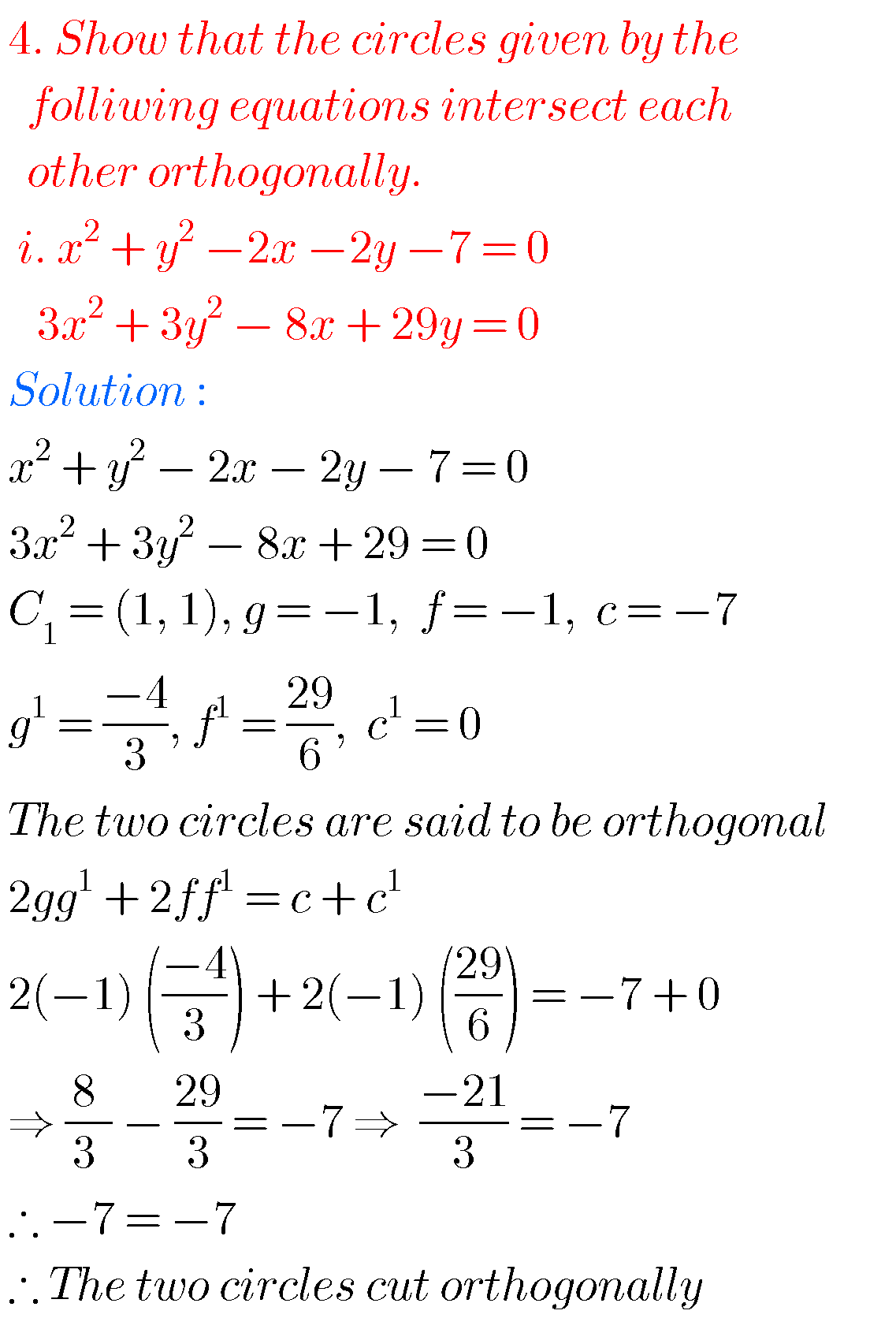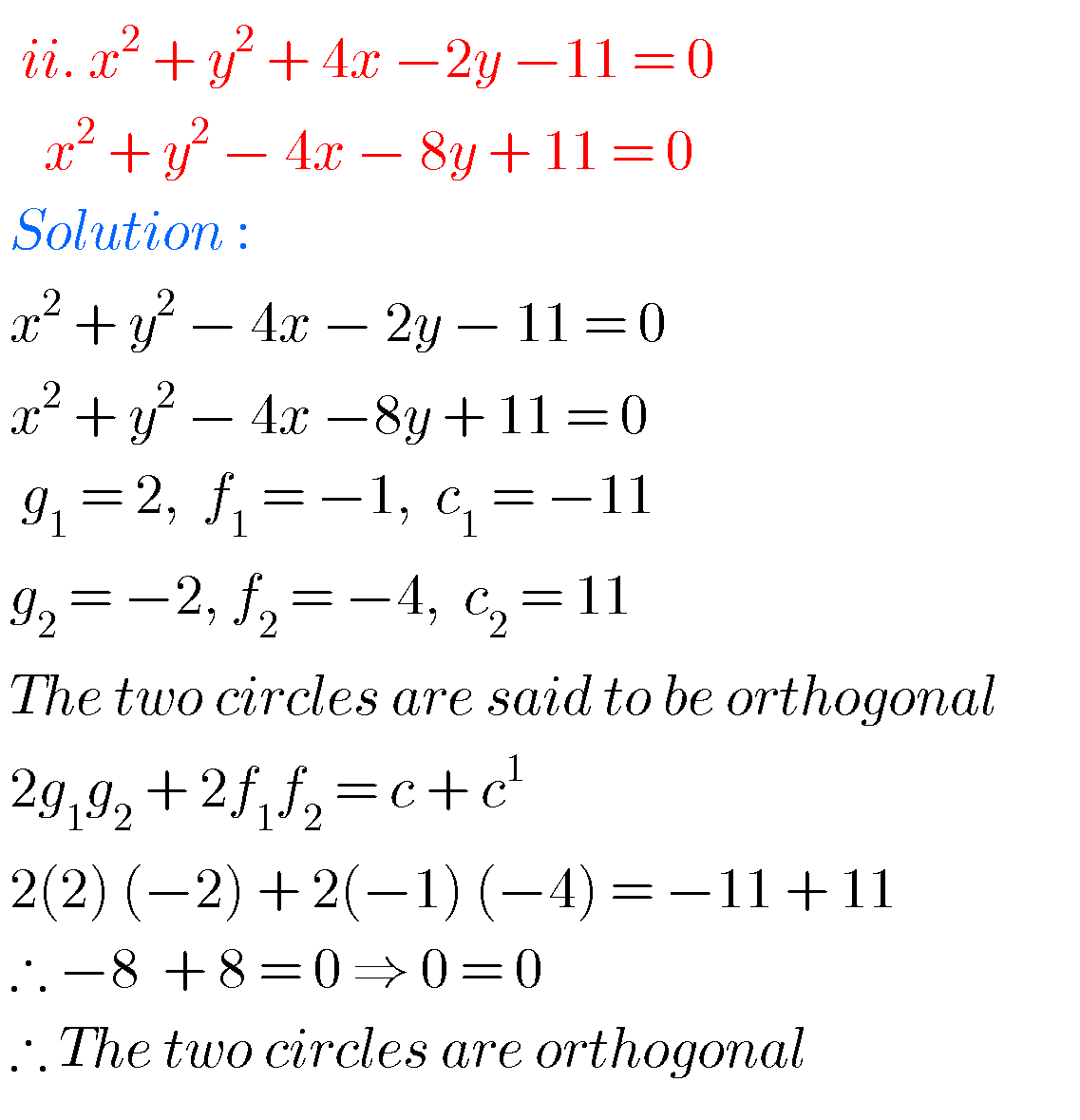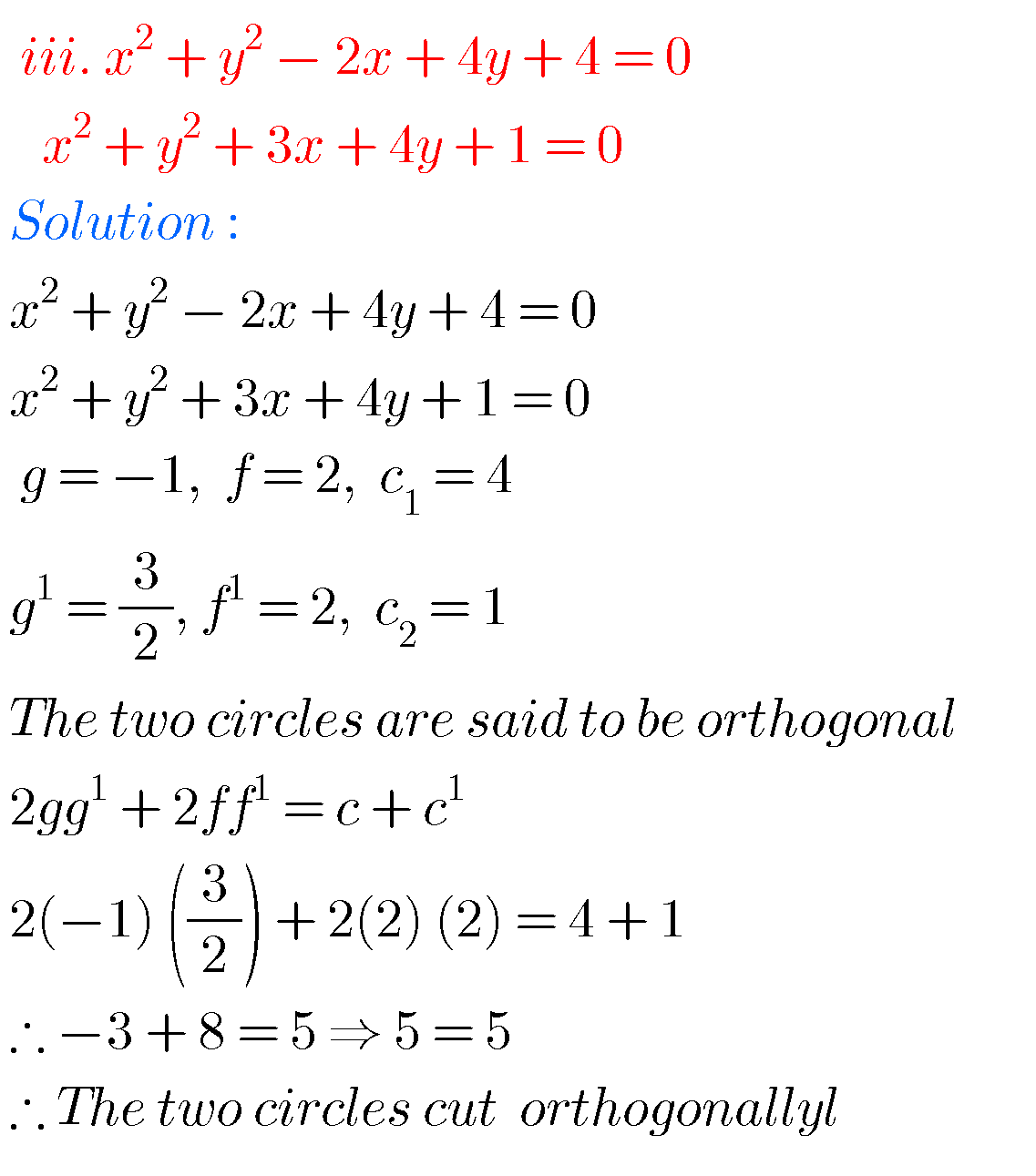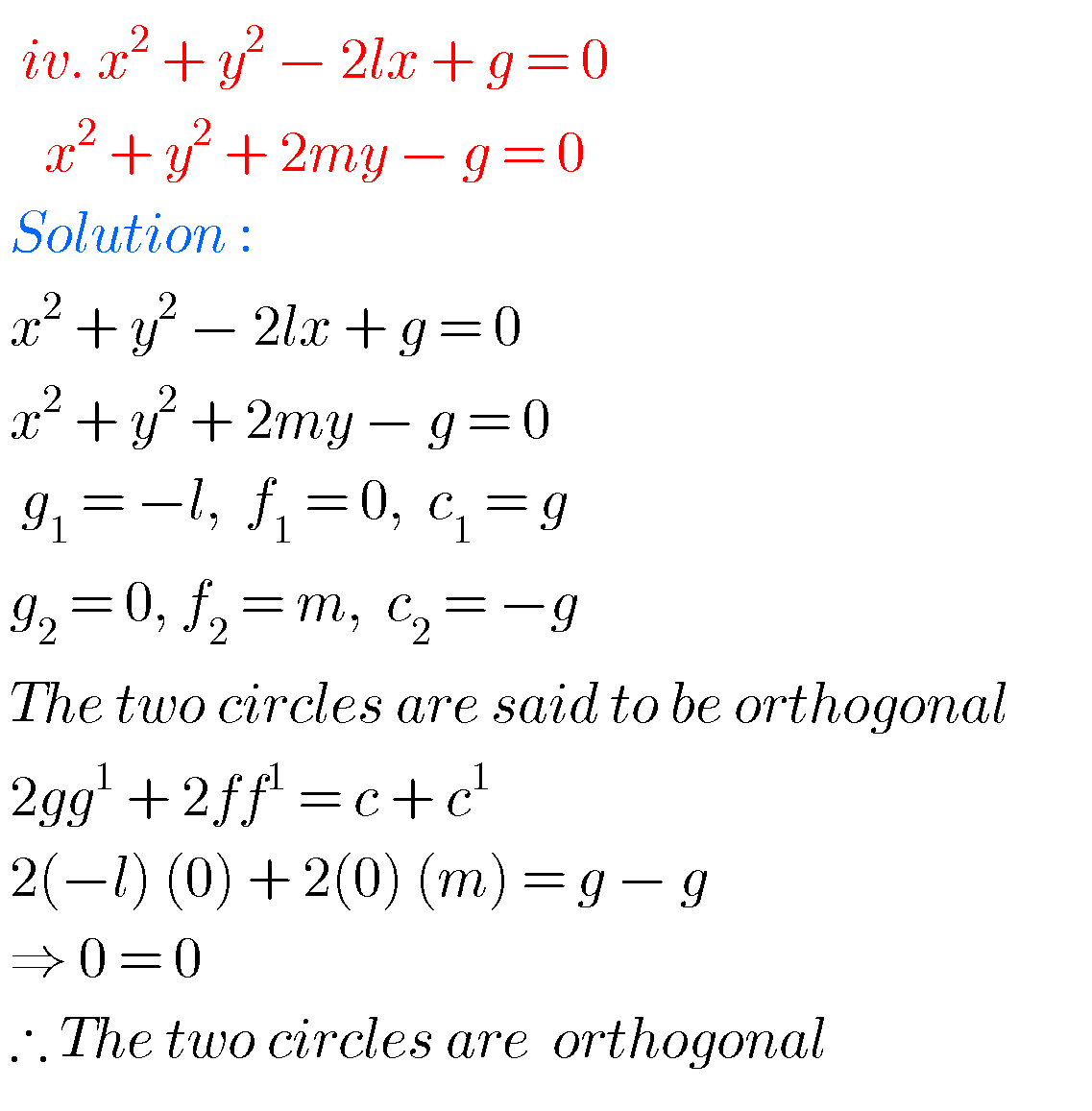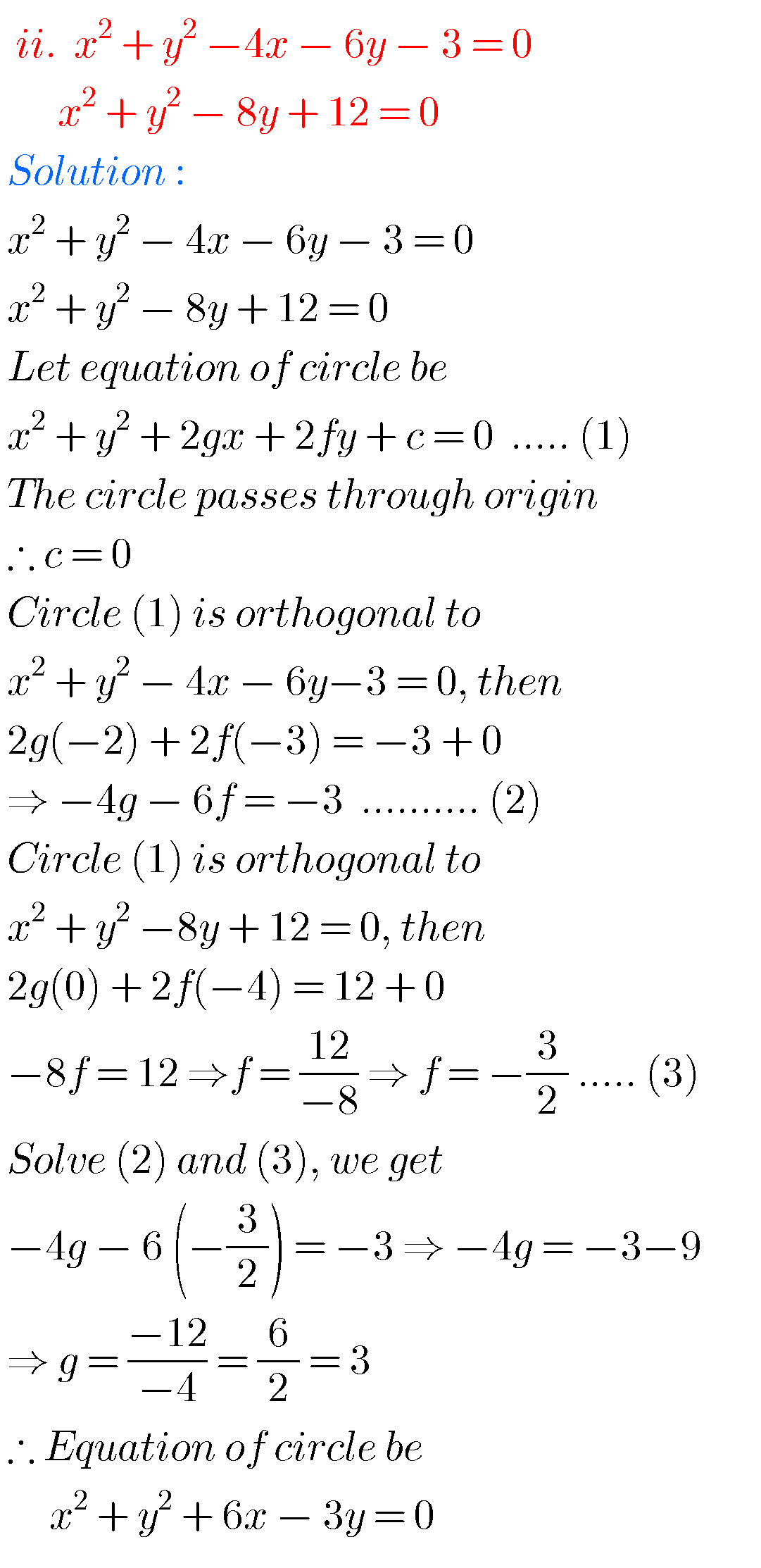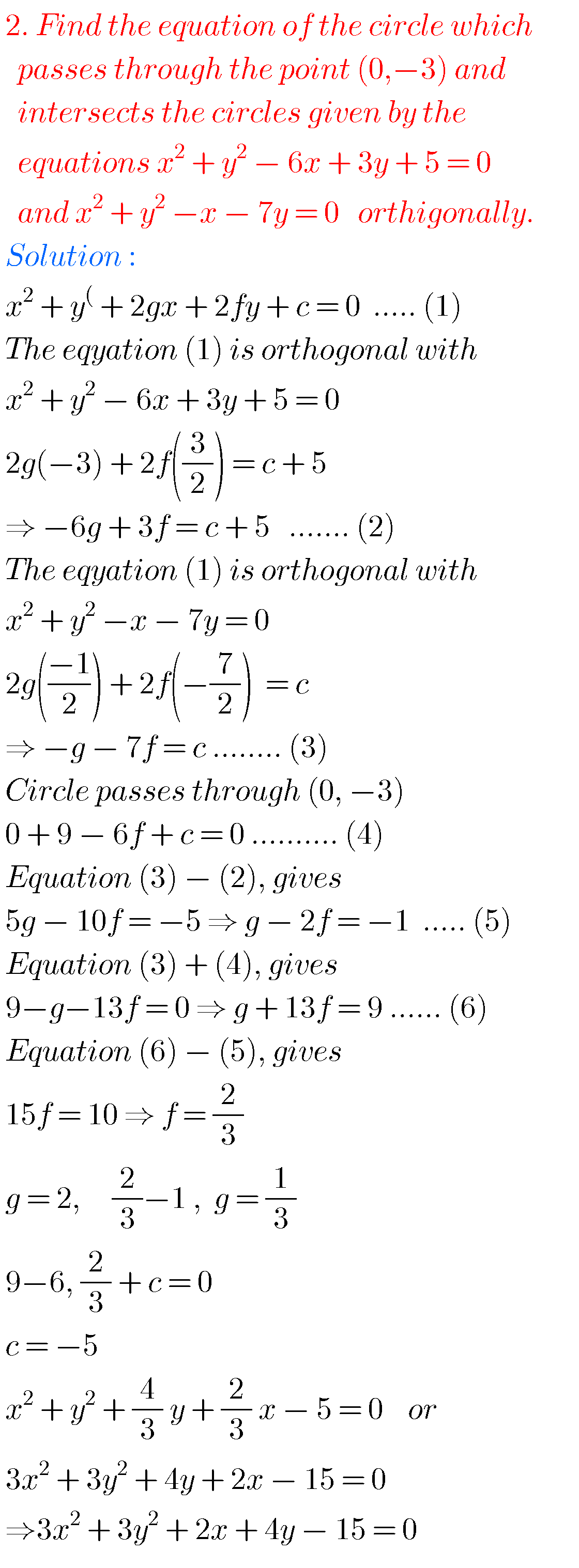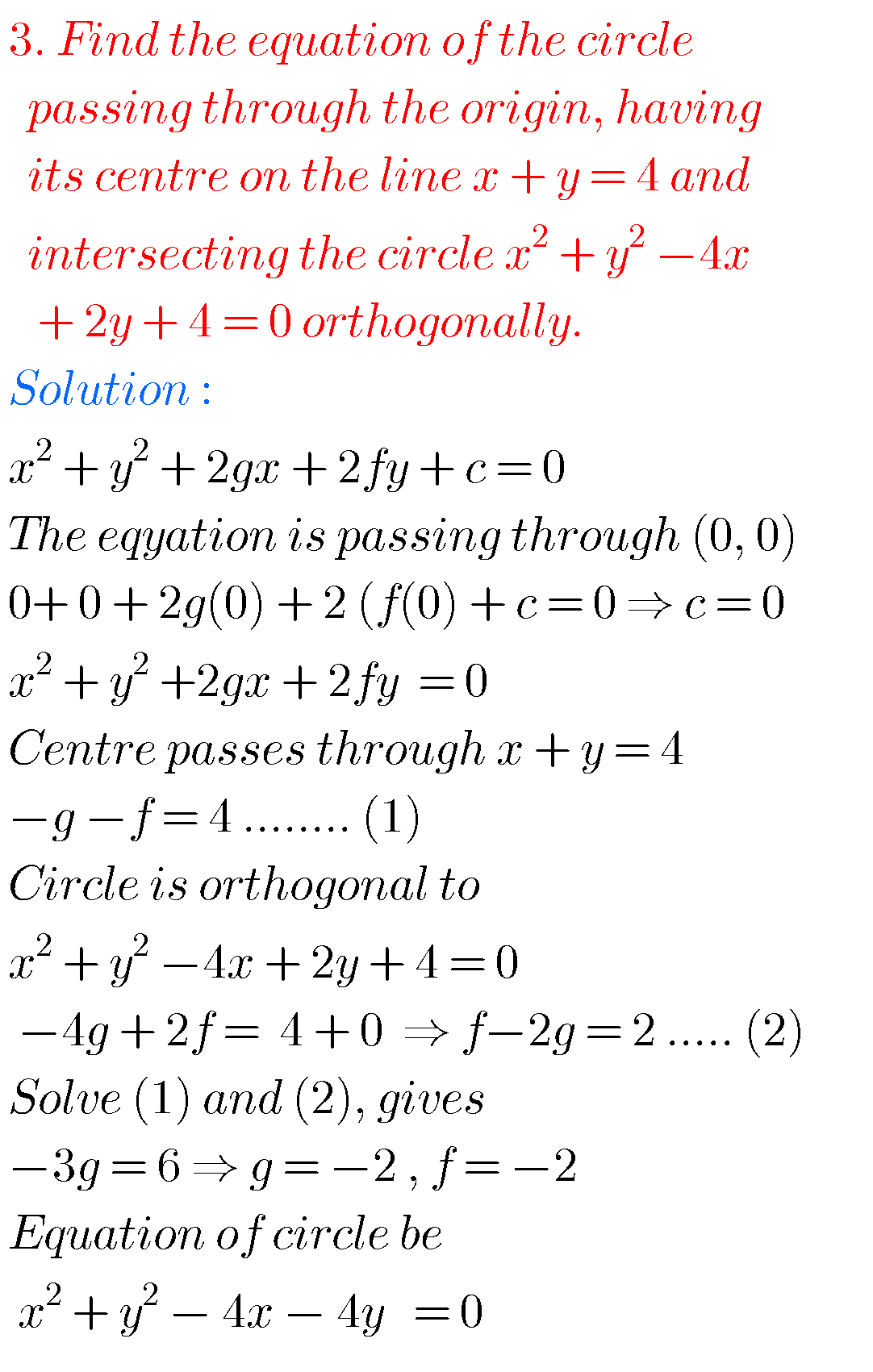## System of Circles solutions Inter maths 2b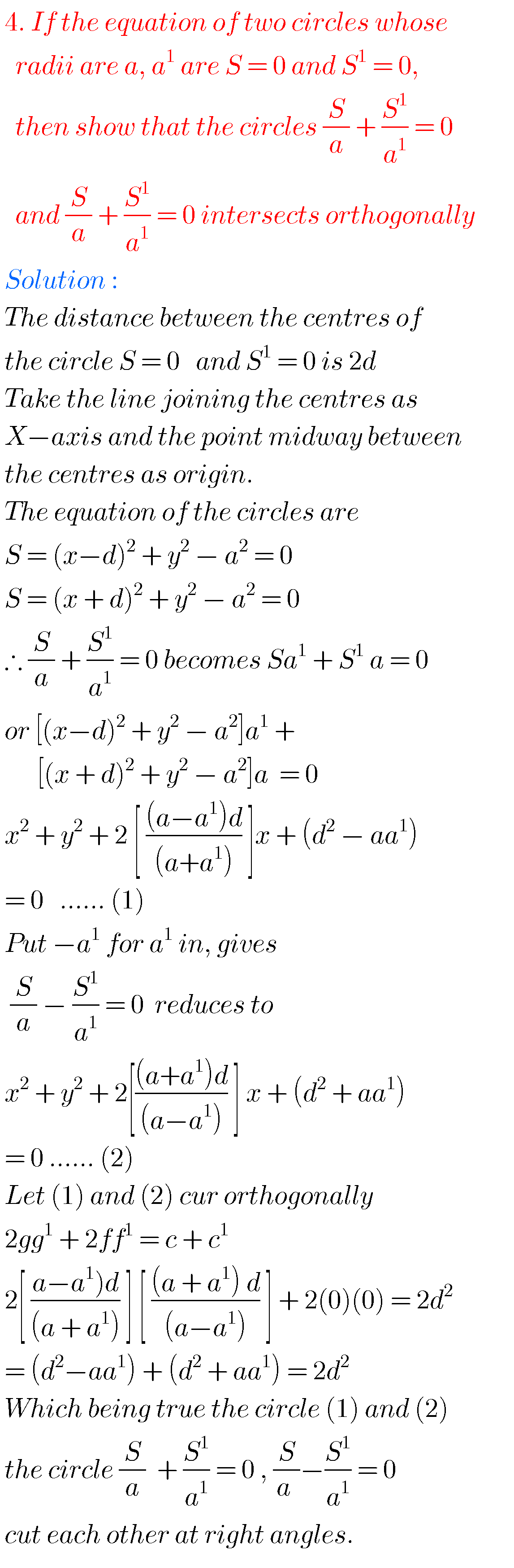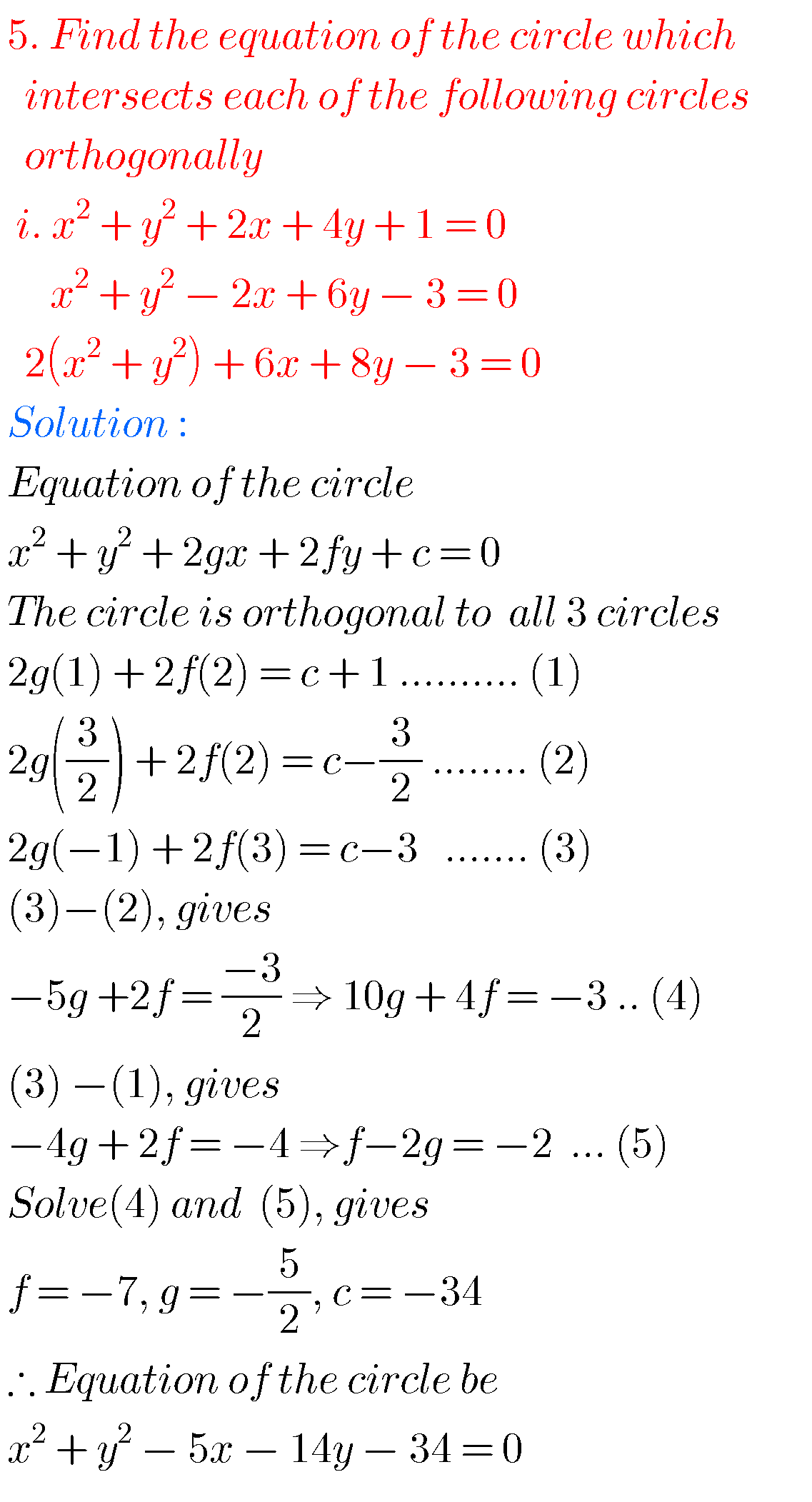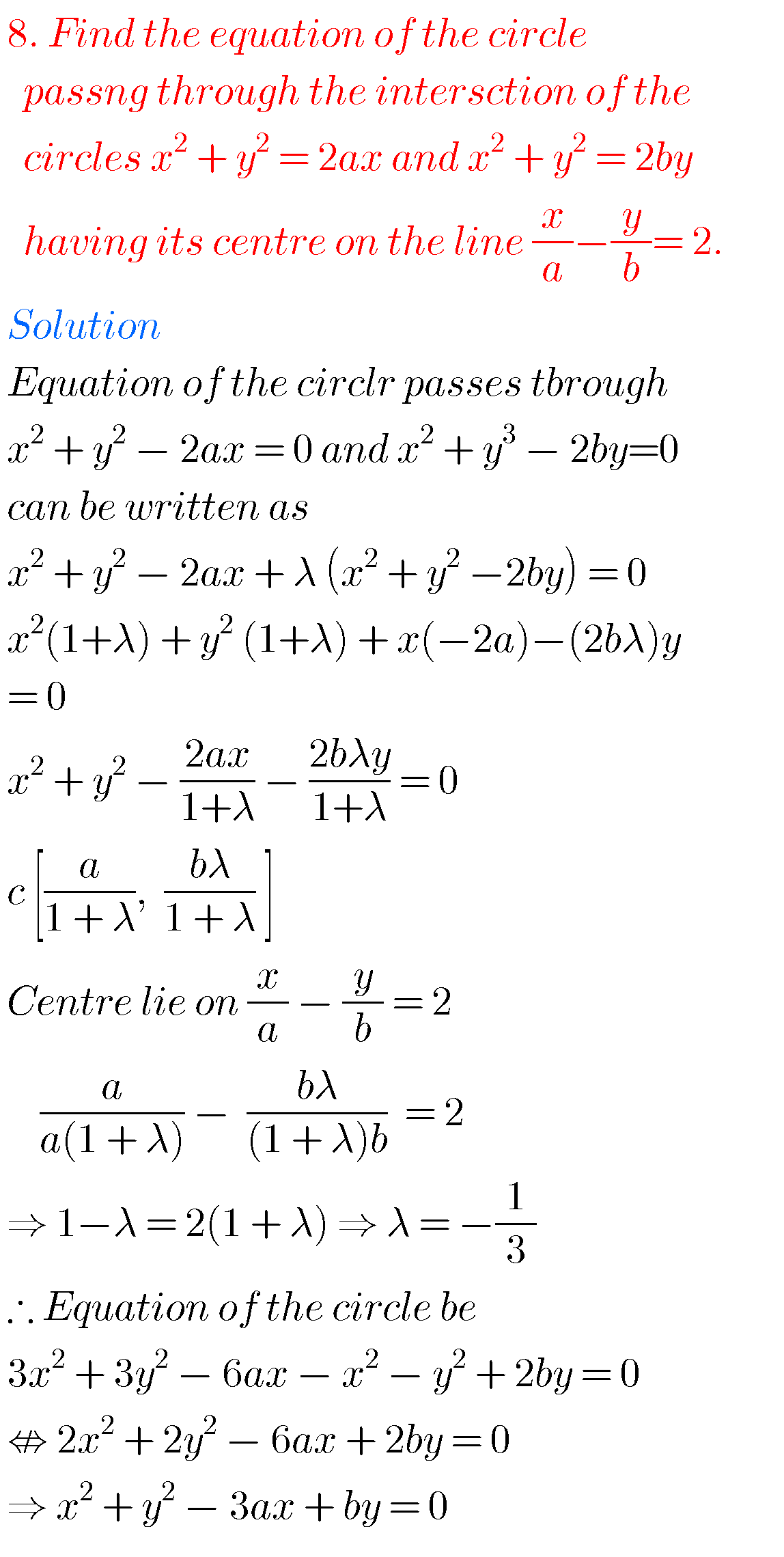## Solutions for System of Circles Inter maths IIB

Exercise 2(b)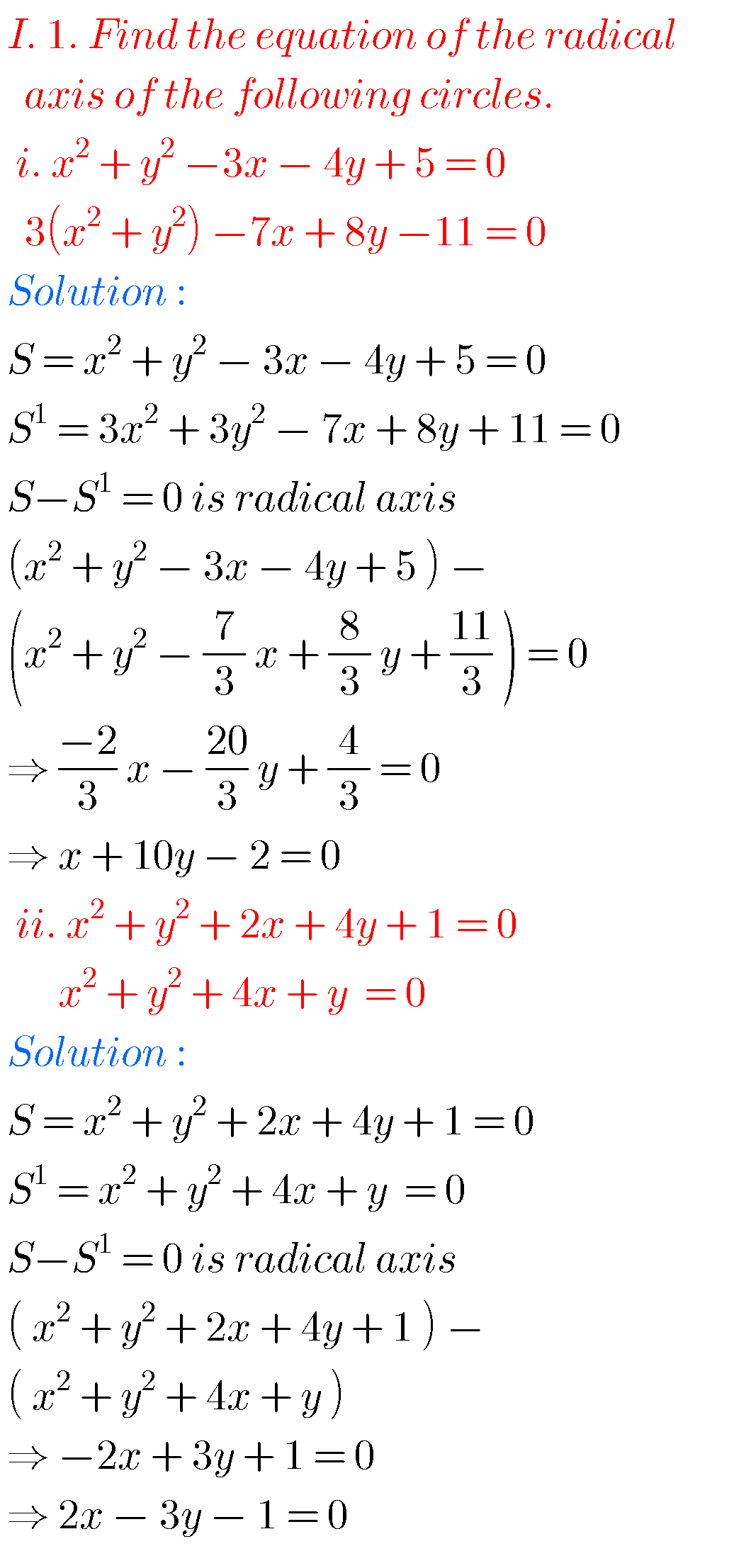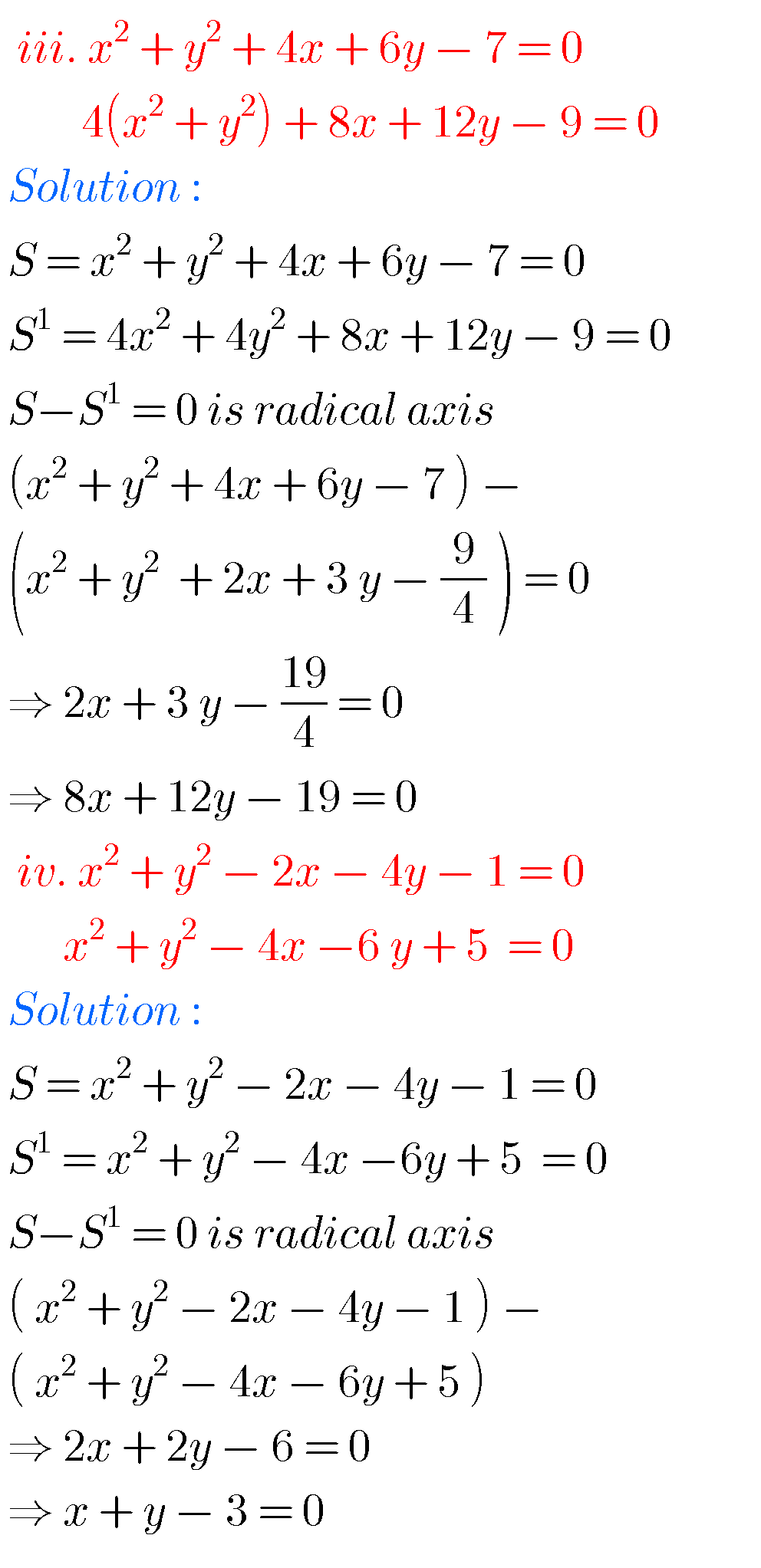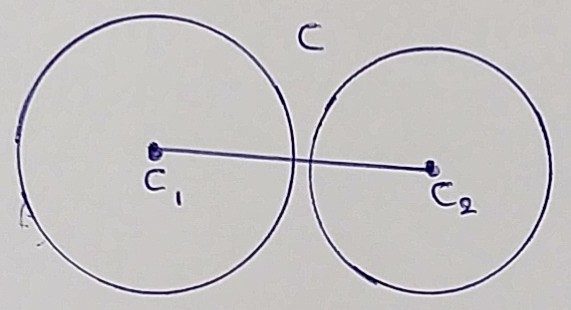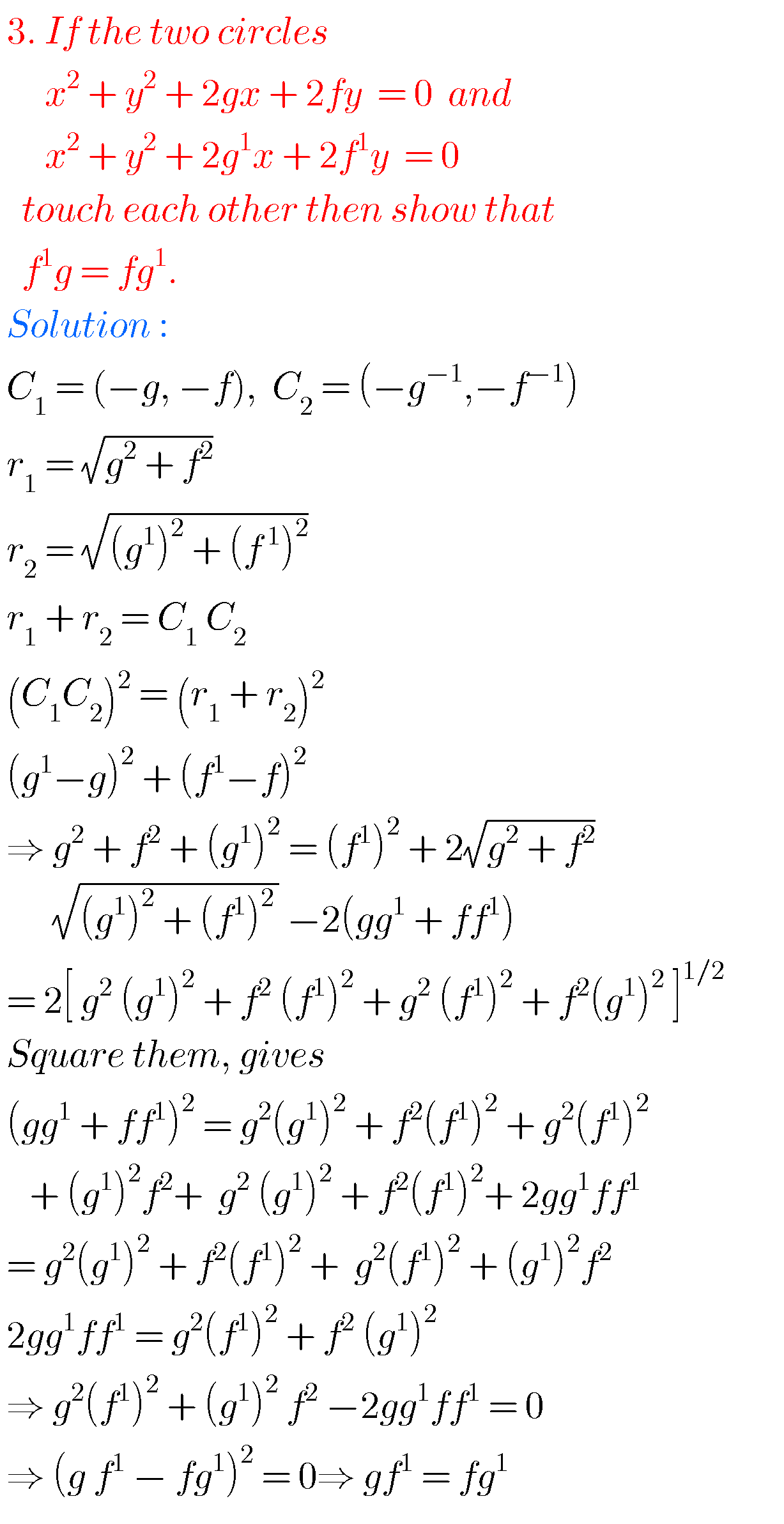### Maths solutions for System of Circles Inter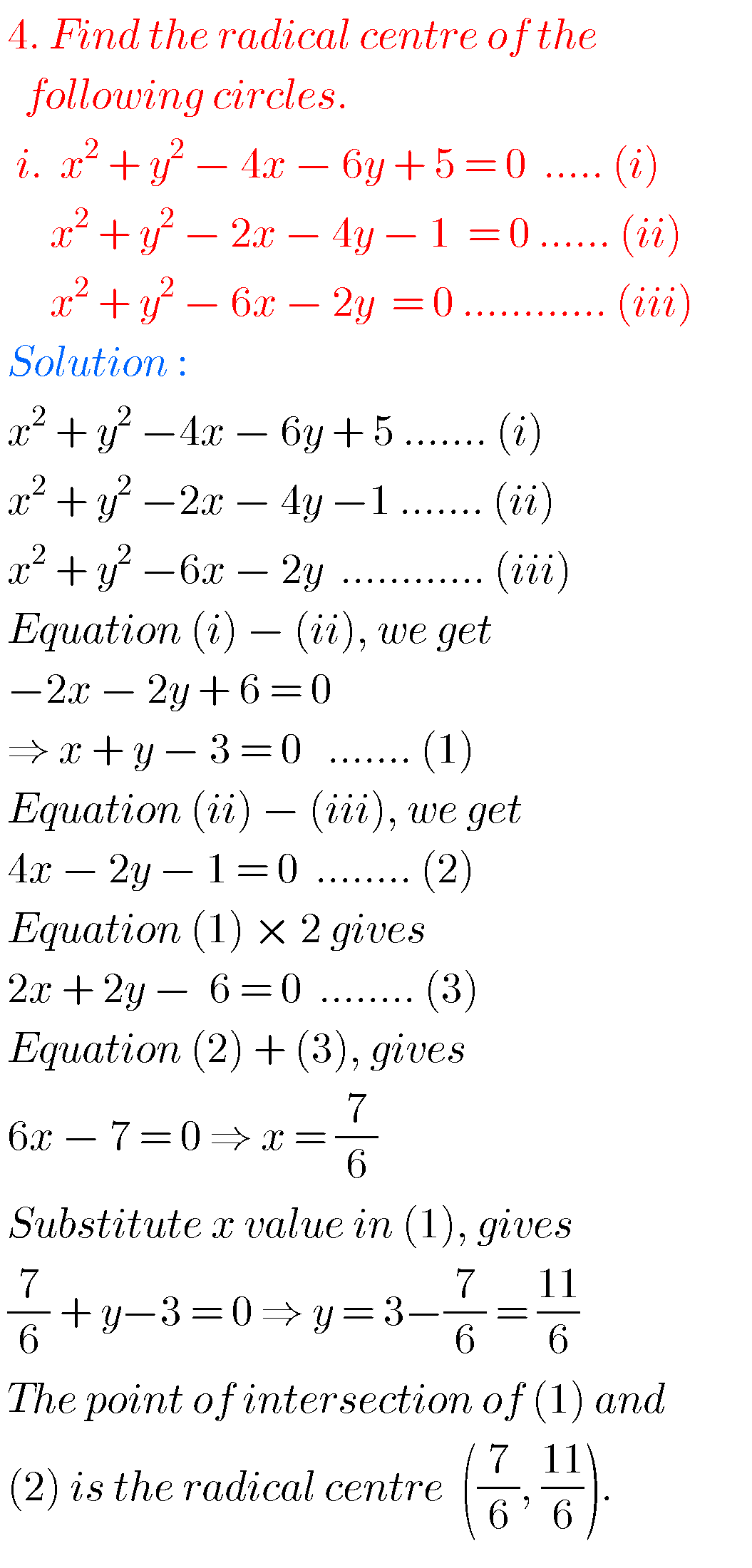### Maths 2a solutions for Syatem of Circles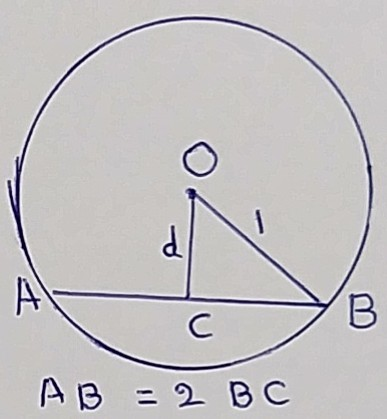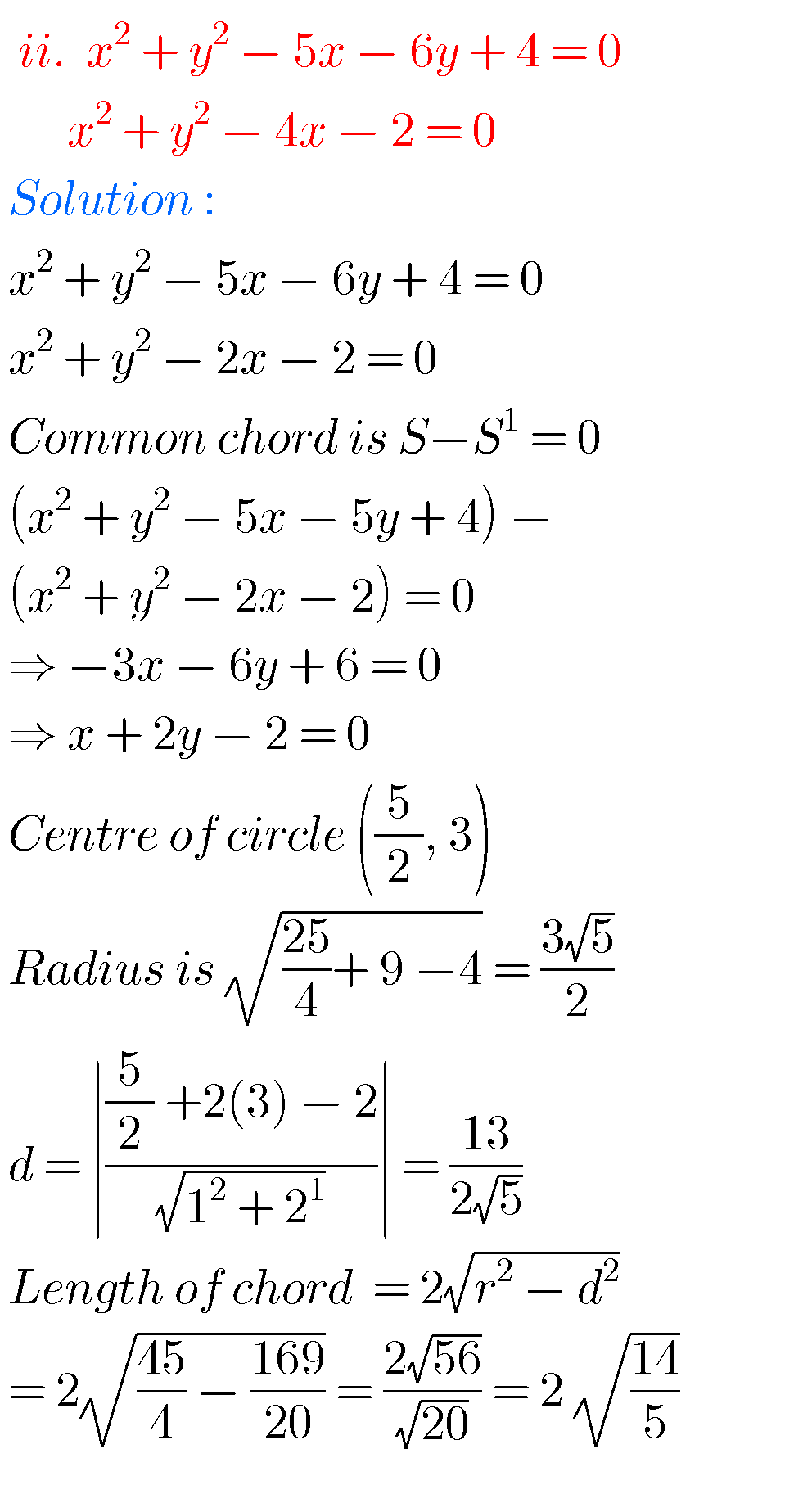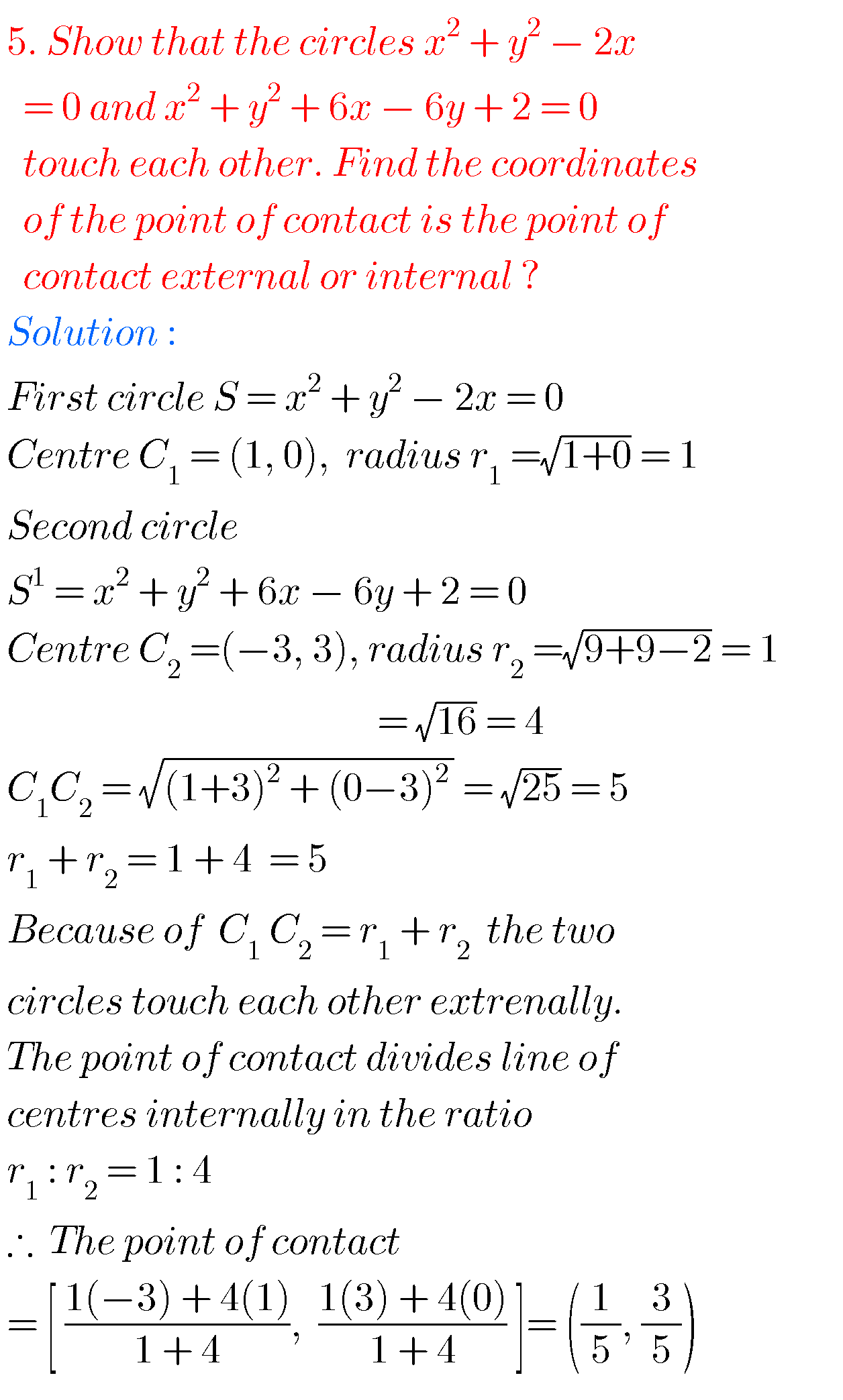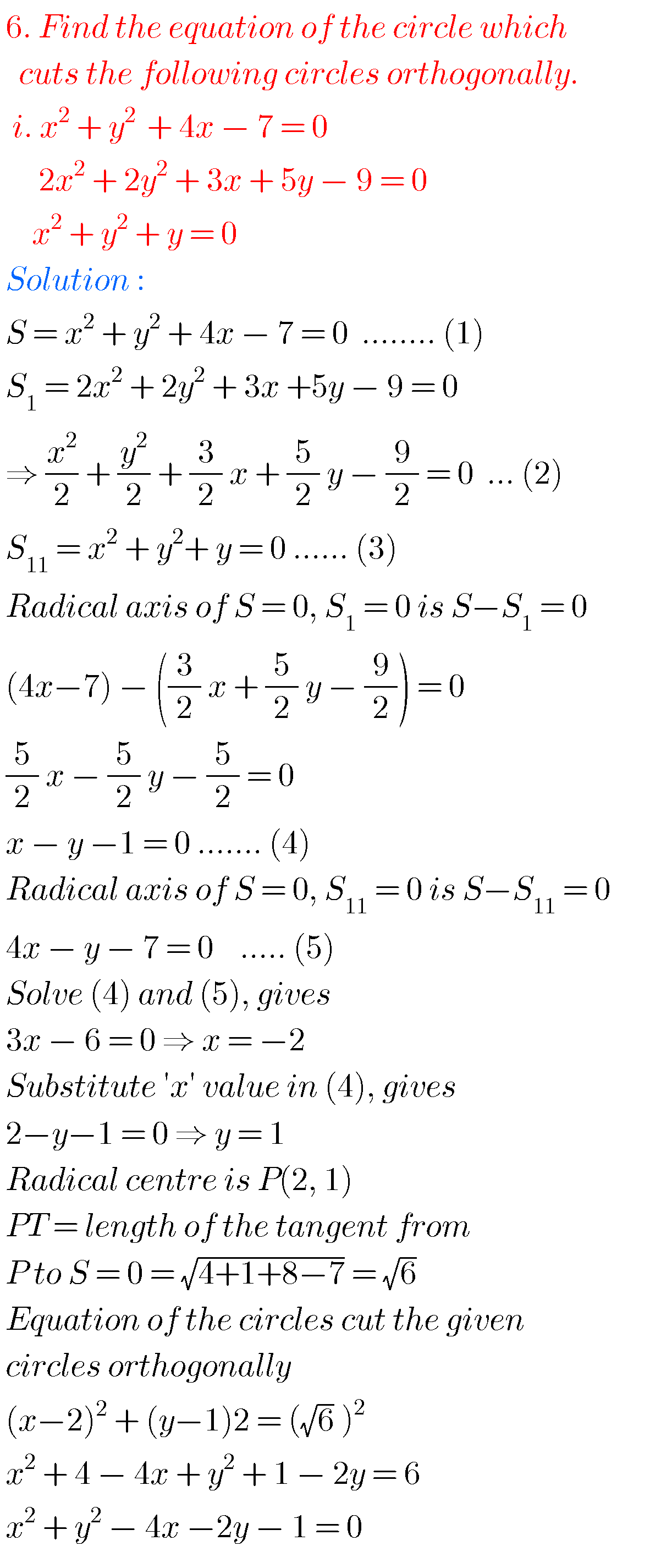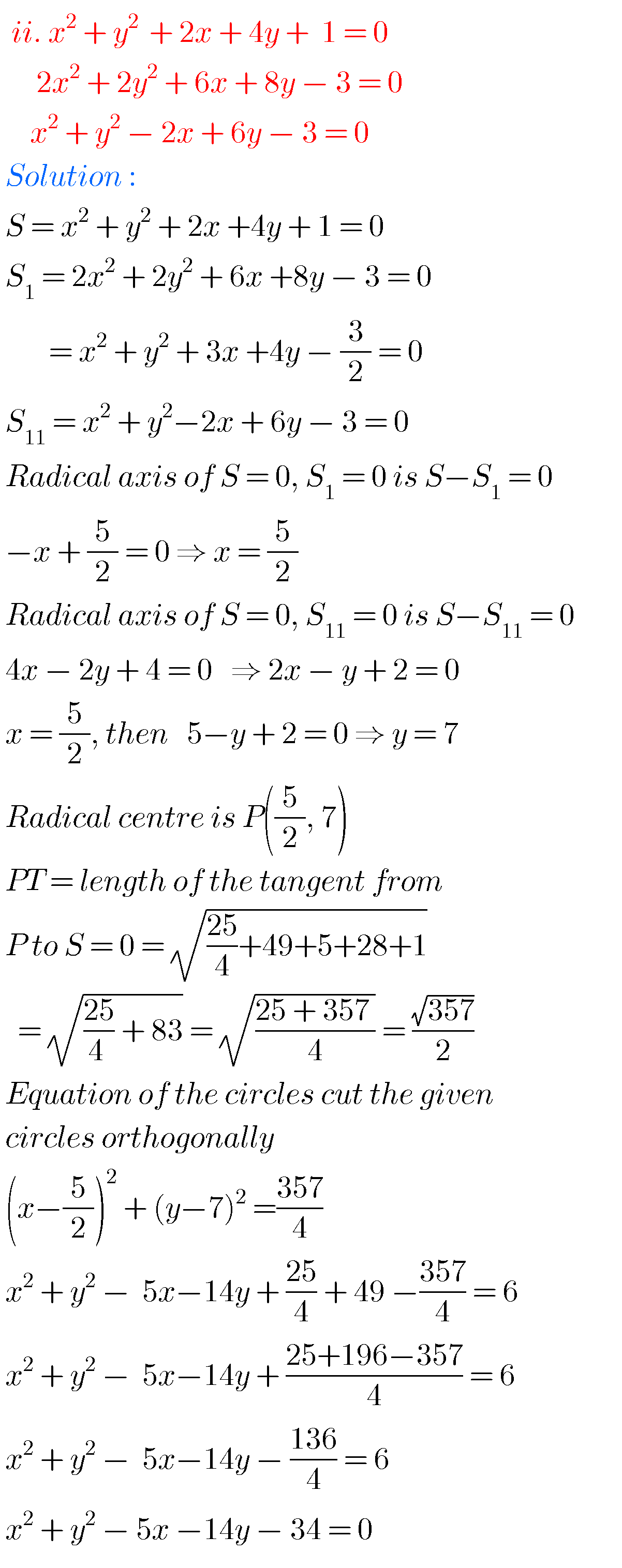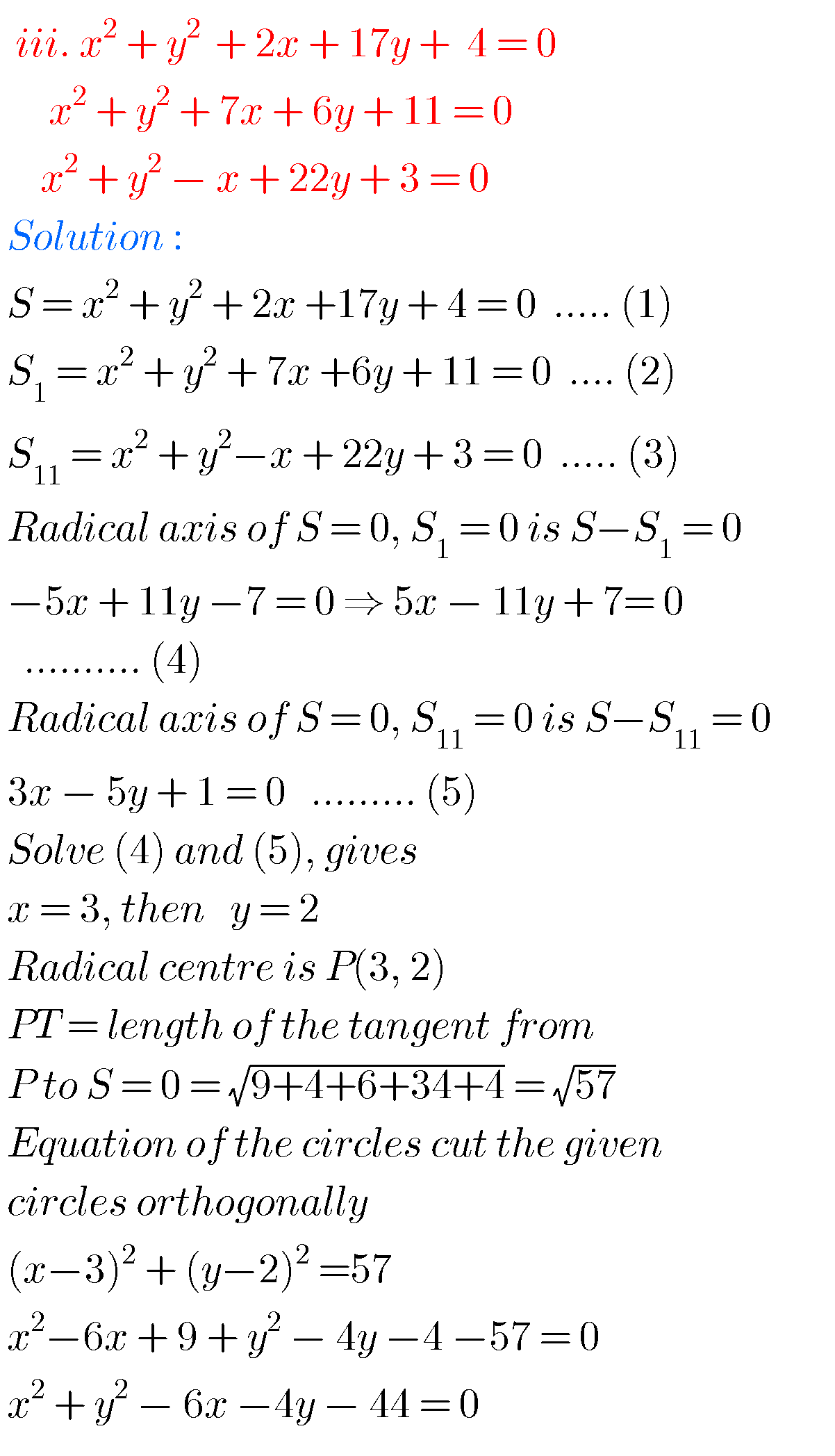Note : Observe the solutions and try them in your own method.

You can see solutions for Inter maths 1A for examination purpose

3. Matrices

You can also see solutions for Inter Maths 1B

1. Locus

You can see solutions for

Ncert solutions for class integers